Verification of Numerical Solutions of the Advection-Diffusion and Burgers Equations | OMICS International
Journal of Applied & Computational Mathematics

# Verification of Numerical Solutions of the Advection-Diffusion and Burgers Equations

Carlos Henrique Marchi1* and Arileide Cristina Alves2

1Department of Mechanical Engineering, Federal University of Paraná, Caixa postal 19040, 81531-980, Curitiba, PR, Brazil

2Núcleo de CiênciasExatas e Tecnológicas, UniversidadePositivo, Curitiba, PR, Brazil

*Corresponding Author:
Carlos Henrique Marchi
Department of Mechanical Engineering
Federal University of Paraná, Caixa postal 19040
81531-980, Curitiba, PR, Brazil
Tel: +55-41-3361-3126
Fax: +55-41-3361-3701
E-mail: [email protected]

Received Date: February 06, 2014; Accepted Date: February 20, 2014; Published Date: February 24, 2014

Citation: Marchi CH, Alves AC (2014) Verification of Numerical Solutions of the Advection-Diffusion and Burgers Equations. J Appl Computat Math 3:154. doi: 10.4172/2168-9679.1000154

Copyright: © 2014 Marchi CH, et al. This is an open-access article distributed under the terms of the Creative Commons Attribution License, which permits unrestricted use, distribution, and reproduction in any medium, provided the original author and source are credited.

Visit for more related articles at Journal of Applied & Computational Mathematics

#### Abstract

The purpose of this work is to verify the asymptotic order of the discretization error of two one-dimensional problems modeled by the advection-diffusion and Burgers equations. The problems are solved using first and second-order accurate spatial approximations, with and without mixing (hybrid scheme), by deferred correction. It was verified that the accuracy of hybrid schemes is equal to that of the lowest-order pure scheme.

#### Keywords

Advection-diffusion; Burgers; CFD; Discretization error; Order of error

#### Introduction

Accurate and reliable numerical solutions depend on the estimation of the numerical error (E), which can be defined as the difference between the exact analytical solution (Φ) of a variable of interest and its numerical solution (φ), i.e.,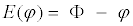(1)

Where E is caused by four sources of error : truncation, iteration, round-off and programming. When the other sources do not exist or are very small compared to the truncation error, E can also be called by discretization error. In practical situations, a numerical solution is obtained because the analytical solution isunknown. Hence, the true value of the numerical error is also unknown and must therefore beestimated. There are two estimators for the discretization error that are widely used with themethods of finite difference and finite volume, both of them based on Richardsonextrapolation. One of them is Richardson estimator, which is given by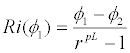(2)

where φ1 and φ2=numerical solutions obtained, respectively, with fine (h1) and coarse (h2) grids, h=grid spacing or distance between every two consecutive nodes of the grid (in this work, h=1/N), N+1=number of nodes in the grid, r=h2/h1=grid refinement ratio, pL=asymptotic order  of the error predicted for each variable of interest based on a priori analyses using a Taylor series .

The accurate estimation (Ri/E→1) of E by means of Equation (2) is only possible if the correct value of pL is used, and h is sufficiently small (h → 0). One way of checking in practice, i.e., through numerical experiments, if the valuededuced a priori for pL is correct is to use the concept of effective order (pE) of the true error, defined by  as: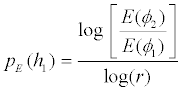(3)

As can be seen in Equation (3), the effective order (pE) is a function of the true error of the variable of interest. Thus, for problems whose analytical solution is known, it can be used to verify a posteriori if, as h → 0, one obtains the asymptotic order (pL) of the discretization error, whichis a theoretical result obtained a priori. In problems whose analytical solution is unknown, the concept of apparent order  can be used to obtain a posteriori the pL of each φ.

In this work, two one-dimensional (1D) problems are solved by the finite difference method with uniform grids. First and second-order accurate numerical approximations in space, and a mixture of the two (hybrid), are used. The objectives of this work are: (1) based on numerical experiments, verify  the true value of the discretization error (E) as a function of the size (h) of the grid for four variables of interest (φ) in each problem; (2) deduce the value of the asymptotic order (pL) of the discretization error of each φ, be it primary or secondary; (3) also based onnumerical experiments, verify if the value of the effective order (pE) of the discretization error tends toward pL when h → 0; and (4) over the discretization error and its order, show the effect caused by the Peclet number, by the numerical approximation used, by the mix factor of hybrid schemes, and by the source term. Another objective is to clarify inconsistent statements found in the literature. For example, Celik and Zhang  state that the asymptotic order of a hybrid scheme is variable. On the other hand, Roache  suggests using the lower order between the two pure schemes. Even in onedimensional problem, Roy  considers that the error is reduced non-monotonically when atleast two schemes with different asymptotic orders are used.

The importance of this work lies in confirming the correct value of the asymptotic order (pL) of the discretization error for some numerical approximations that are very common in the method of finite difference. This will allow the Richardson estimator and its variants to be used correctly, since they depend directly on the value of pL, as can be seen in Equation (2). The correct value of the asymptotic order is also important to use the repeated Richardson extrapolation efficiently . Another contribution is to show the behavior of the error with the deferred correction scheme [9-11]. In addition, we intend to clarify the aforementioned open questions in the literature. One-dimensional problems are used here for the following reasons: (1) the possibility of using highly refined grids in one direction up to an order of millions of nodes, which enables one to verify asymptotic behaviors and thus unequivocally prove the value of practical pL; (2) due to the speed in obtaining each solution, a large number of tests can be performed, facilitating systematic studies of several parameters; and (3) it is presumed that the onedimensional results are applicable to two and three dimensions. The work is divided as follows: sections II and III address two one-dimensional problems in a steady state, the advectiondiffusion and Burgers equations, and section IV reports the conclusion of this work.

Mathematical model

Starting from the thermal energy conservation equation, considering a steady state 1D incompressible fluid flow without heat generation and viscous dissipation, and consideringthat the properties and velocities are constant in a continuous medium, one obtains theadvection-diffusion equation: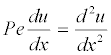(4)

where Pe=the Peclet number, x=coordinate direction, and u=temperature. The boundary conditions are of the Dirichlet type: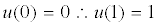(5)

The variables of interest, i.e., the variables for which the numerical solution is obtainedand its discretization error and effective order are verified, are:

(a) Temperature (u) at x=½: the principal variable of the problem, which is obtained fromthe solution of Equation (4). Its analytical solution is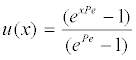(6)

(b) Mean temperature (U): a global variable obtained from the following definition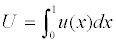(7)

Its analytical solution is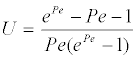(8)

(c) Inclination (I): a local variable obtained from the following definition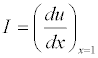(9)

Its analytical solution is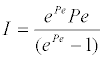(10)

(d) The mean of the l1 norm of the discretization error (L), defined mathematically by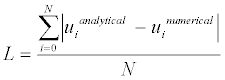(11)

where i represents each of the nodes of the grid. Its analytical solution has a null value.

Numerical model

The numerical model is characterized by the use of the finite difference method , uniform grids and the TDMA (Tri Diagonal Matrix Algorithm) method to solve the system ofequations. The programming language used is FORTRAN 95 with quadruple precision. The diffusive term (second-order derivative) of Equation (4) was approximated with the centraldifferencing scheme (CDS). In the case of the advective term (first-order derivative), three approximations were used: (1) CDS, (2) first-order upwind differencing scheme (UDS), and (3) β scheme, which is a hybrid UDS and CDS scheme by means of the deferred correction of [10,11], i.e.,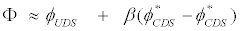(12)

Where Φ=exact value of the advective term, φ=numerical approximation in the currentiteration, φ*=numerical approximation in the previous iteration, and β=mix factor whosevalue varies from 0 (UDS) to 1 (CDS). In the deferred correction scheme, all the terms that involve β are considered source terms, remaining in the independent term of the system of equations. The coefficients of the system of equations are the same as those of the pure UDS scheme. The matrix of coefficients is of the tridiagonal type. For the UDS and CDS schemes, the TDMA solves the system directly. In the case of the β scheme, due to the second term of Equation (12), the solution is iterative; in this case, the initial estimation is equal to the analyticalsolution. Details about the above described numerical model and those of the next sections aregiven by [4,11]. The variable of mean temperature (U) was obtained through the trapezoidal rule [12,13], andthe variable of inclination (I) was obtained with the UDS-2 scheme, i.e., the second-order UDS .

Estimation of the asymptotic order (pL)

Based on the Taylor series and following the procedure of , the truncation error (ε) of the discretized differential equation (EDD) at each i node of the grid is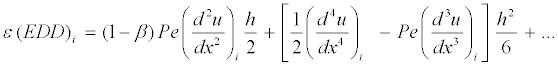(13)

By definition, the asymptotic order (pL) is the lowest exponent of the error, whose termprevails when h → 0. For uniform grids, it is known  that the pL of the discretization errorof the unknown of the differential equation, E(u), is equal to the pL of ε (EDD). Therefore, for u, one has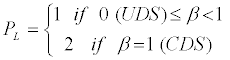(14)

For U, obtained by means of the trapezoidal rule, one has  pL=2. This is the sameresult as for I, obtained with the UDS-2 scheme . But these values are valid for thesituation in which ui has no error, i.e., using the analytical solution in each node. In the case ofsecondary variables (φ), i.e., variables that depend on the primary variable (u), in other words, the unknown in the differential equation, due to error propagation, the pL of the discretizationerror (E) is given by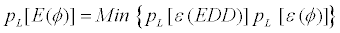(15)

where Min=minimum value between the two arguments, and ε(φ)=truncation error of a numerical approximation φ. Hence, the result of Equation (14) is also valid for U, I and L.

Numerical results

The numerical solution of the four variables of interest was obtained with grids of 3, 5, …up to 67,108,865 nodes, which correspond to h=½, ¼, ... down to ≈ 1.49×10-8 m. In thesolution of Equation (4), the schemes UDS, CDS and β=0.999 were used for Pe=1 and 10. Acomputer program was implemented in FORTRAN 95 language, version 9.1 of Intel, usingquadruple precision (Real*16). The simulations were performed on a microcomputerequipped with an Intel processor (Xeon Quad Core X5355 2.66 GHz), 16 GB RAM andWindows XP 64 bits operational system. The maximum CPU time was 9 minutes for the β scheme with eight iterations, a sufficient number to reach the machine round-off error. Figure 1 shows the modulus of the discretization error (E) of the four variables as a function of the h grid used. Considering the modulus of E, one can see that for a relativelylarge h, in general |E(β)| ≈ |E(CDS)| < |E(UDS)|. And for h → 0, |E(CDS)| < |E(β)| < |E(UDS)|. Moreover, for the same scheme, in general |E(Pe=1)| < |E(Pe=10)|. Figure 2 shows the effetive order (pE) of the error of the four variables as a function ofthe h grid used. All the results of pE presented in this work were obtained with Equation (3) and a refinement ratio (r) equal to 2. Note, in Figure 2, that:

1) In the coarser grids, as expected , the values of the effective order (pE) can besignificantly different from those of the asymptotic order (pL), presenting negative or evenundefined values.

2) For h → 0, pE→ pL as predicted by Equation (14) for the three schemes (UDS, CDS and β) and the four variables of interest, even for β scheme with its value equal to 0.999.

3) For temperature and L, in the case of β scheme, pE≈ pL(CDS) in the coarser grids. Uponreducing h, there is an interval in which pE is undefined. And lastly, for h → 0, pE→ pL(UDS).

5) For mean temperature, in the case of β scheme, pE ≈ pL(UDS) in almost all the gridsexcept in the coarsest ones.

6) Although for β scheme, pE → pL(UDS) for h → 0, its error may be significantly smallerthan that of the UDS, depending on the value of β (Figure 1).

The literature contains the following inconsistent statements concerning the numerical solution of the advection-diffusion equation: (i) Celik and Zhang  state that the asymptotic order of a hybrid scheme is variable; (ii) Roache  suggests using the lower order between the two pure schemes; and (iii) even in one-dimensional problems, Roy  considered that the error is reduced non-monotonically when at least two schemes with different asymptotic orders are used. Therefore, based on the theoretical and numerical results presented in this section, the statement of Roache  is correct, that of Celik et al.  is incorrect, and that of Roy  is correct depending on the variable of interest.

#### Burgers Equation

Mathematical model

Starting from the equation of conservation of the momentum, considering a continuous medium of incompressible fluid with constant properties and steady state 1D laminar flow, one obtains the Burgers equation with a source term given by(16)

where Re=Reynolds number, x=coordinate direction, and u=velocity. The boundaryconditions are of the Dirichlet type, given by Equation (5).

The variables of interest, i.e., the variables for which the numerical solution is obtained and its discretization error and effective order are verified, are:

(a) Velocity (u) at x=½: obtained from the solution of Equation (16). Its analytical solution is given by Equation (6) with Pe=1.

(b) Mean velocity (U): defined by Equation (7). Its analytical solution is given by Equation (8) with Pe=1

(c) Inclination (I): defined by Equation (9). Its analytical solution is given by Equation (10) with Pe=1.

(d) The mean of the l1 norm of the discretization error (L), defined by Equation (11). Its analytical solution has a null value.

Numerical model

The numerical model is the same as the one adopted for the advection-diffusion equation. The only difference is that the u that multiplies the advective term is incorporated into the coefficients of the system of equations to linearize the nonlinear differential equation. In this case, the solution of the system of equations is iterative with TDMA for the three schemes used in the advective term: UDS, CDS and β.

Estimation of the asymptotic order (pL)

Based on the Taylor series and following the procedure of , the truncation error (ε) of the discretized differential equation (EDD) at each i node of the grid is 2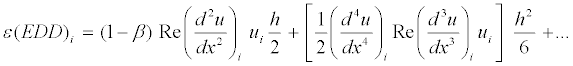(17)

Therefore, the same result of Equation (14) applies to u, U, I and L.

Numerical results

The numerical solution of the four variables of interest was obtained with grids of 3, 5, ... up to 33,554,433 nodes, which correspond to h=½, ¼, ... down to ≈2.98×10-8 m. In the solution of Equation (16), the schemes UDS, CDS and β=0.99 were used for Re=5. The CPU time was at most 1 h 12 min for β scheme with 100 iterations, a sufficient number to reach the machine round-off error. Figure 3 shows the modulus of the discretization error (E) of the four variables as a function of the h grid used. Considering the modulus of E, one can see that for a relatively large h, in general |E(β)| ≈ |E(CDS)| < |E(UDS)|, and for h → 0, |E(CDS)| < |E(β)| < |E(UDS)|. Figure 4 shows the effective order (pE) of the error of the four variables of interest as a function of the h grid used. In this figure, note that:

1) In the coarser grids, as expected , the values of the effective order (pE) can differsignificantly from those of the asymptotic order (pL), presenting negative or evenundefined values.

2) For h → 0, pE→ pL, as predicted by Equation (14) for the three schemes (UDS, CDS and β) and the four variables of interest, even for β scheme with its value of 0.99.

3) For velocity and L, in the case of β scheme, pE≈ pL(CDS) in the coarsest grids. Uponreducing h, there is an interval in which pE is undefined. And lastly, for h → 0, pE→pL(UDS).

4) For the mean velocity and the inclination, in the case of β scheme, pE≈ pL(CDS) in thecoarsest grids. Upon reducing h, there is an interval in which pE varies monotonicallydown to h → 0, pE → pL(UDS).

5) Although for β scheme, pE→ pL(UDS) for h → 0, its error may be significantly smallerthan that of the UDS, depending on the value of β (Figure 3).

The same comments made in the last paragraph of the section about the advectiondiffusionequation also apply to the results of the problem in this section with respect to Burgers equation.

#### Conclusion

This work found principally that:

1) For h → 0, in all the cases, pE→ pL, as predicted by Equation (14), and monotonically for asufficiently small h.

2) The pL of a hybrid scheme is equal to the pL of the pure scheme of the lowest order.

3) For hybrid schemes, the value of the error modulus falls within those of the pure schemes,except in very coarse grids. The proximity of the value of the error modulus between thehybrid scheme and the scheme of the highest order depends on the value employed for the β factor.

4) The value of the parameters Pe and β can affect the value of the error significantly.

#### Acknowledgements

The authors would like to acknowledge the financial support provided by CNPq (Conselho Nacional de Desenvolvimento Científico e Tecnológico, Brazil), CAPES (Coordenação de Aperfeiçoamento de Pessoal de Nível Superior, Brazil), Fundação Araucária (Paraná, Brazil) and the Brazilian Space Agency (AEB), by the Uniespaço Program. The firstauthor is supported by a CNPq scholarship. The authors would also like to acknowledge the suggestions provided by the reviewers.

#### References

Select your language of interest to view the total content in your interested language

### Article Usage

• Total views: 12570
• [From(publication date):
March-2014 - Dec 12, 2019]
• Breakdown by view type
• HTML page views : 8739Can't read the image? click here to refresh